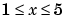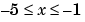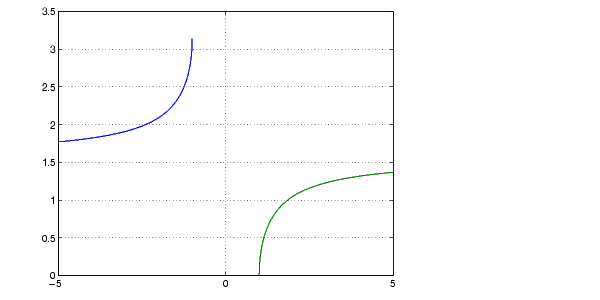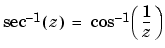MATLAB Function Referenceasec

Inverse secant, result in radians

Syntax

• ```Y = asec(X)
```

Description

```Y = asec(X) ``` returns the inverse secant (arcsecant) for each element of `X`.

The `asec` function operates element-wise on arrays. The function's domains and ranges include complex values. All angles are in radians.

Examples

Graph the inverse secant over the domainsand.

• ``````x1 = -5:0.01:-1;
``````x2 = 1:0.01:5;
``````plot(x1,asec(x1),x2,asec(x2)), grid on
``````

Definition

The inverse secant can be defined as

•Algorithm

`asec` uses FDLIBM, which was developed at SunSoft, a Sun Microsystems, Inc. business, by Kwok C. Ng, and others. For information about FDLIBM, see http://www.netlib.org.

See Also

`asecd`, `asech`, `sec`

© 1994-2005 The MathWorks, Inc.